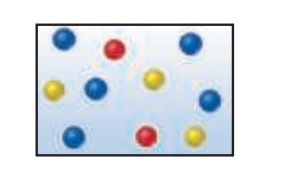×
Get Full Access to Chemistry: The Central Science - 14 Edition - Chapter 10 - Problem 10.7
Get Full Access to Chemistry: The Central Science - 14 Edition - Chapter 10 - Problem 10.7

×

# ?The accompanying drawing represents a mixture of three different gases. (a) Rank the three components in order of increasing partial pressurISBN: 9780134414232 1274

## Solution for problem 10.7 Chapter 10

Chemistry: The Central Science | 14th Edition

• Textbook Solutions
• 2901 Step-by-step solutions solved by professors and subject experts
• Get 24/7 help from StudySoup virtual teaching assistantsChemistry: The Central Science | 14th Edition

4 5 1 382 Reviews
17
2
Problem 10.7

The accompanying drawing represents a mixture of three different gases.

(a) Rank the three components in order of increasing partial pressure.

(b) If the total pressure of the mixture is 1.40 atm, calculate the partial pressure of each gas. [Section 10.6]Step-by-Step Solution:

Step 1 of 5) The acceptable maximum concentrations of toxic or carcinogenic substances in the environment are often expressed in ppm or ppb. For example, the maximum allowable concentration of arsenic in drinking water in the United States is 0.010 ppm; that is, 0.010 mg of arsenic per liter of water. This concentration corresponds to 10 ppb.Concentration expressions are often based on the number of moles of one or more components of the solution. Recall from Section 10.6 that the mole fraction of a component of a solution is given by, the symbol X is commonly used for mole fraction, with a subscript to indicate the component of interest. For example, the mole fraction of HCl in a hydrochloric acid solution is represented as XHCl. Thus, if a solution contains 1.00 mol of HCl 136.5 g2 and 8.00 mol of water 1144 g2, the mole fraction of HCl is Mole fractions have no units because the units in the numerator and the denominator cancel. The sum of the mole fractions of all components of a solution must equal 1. Thus, in the aqueous HCl solution,. Mole fractions are very useful when dealing with gases, as we saw in Section 10.6, but have limited use when dealing with liquid solutions. Recall from Section 4.5 that the molarity (M) of a solute in a solution is defined.

Step 2 of 2

##### ISBN: 9780134414232

The full step-by-step solution to problem: 10.7 from chapter: 10 was answered by , our top Chemistry solution expert on 10/03/18, 06:29PM. This full solution covers the following key subjects: . This expansive textbook survival guide covers 29 chapters, and 2820 solutions. This textbook survival guide was created for the textbook: Chemistry: The Central Science, edition: 14. Chemistry: The Central Science was written by and is associated to the ISBN: 9780134414232. The answer to “?The accompanying drawing represents a mixture of three different gases. (a) Rank the three components in order of increasing partial pressure. (b) If the total pressure of the mixture is 1.40 atm, calculate the partial pressure of each gas. [Section 10.6]” is broken down into a number of easy to follow steps, and 41 words. Since the solution to 10.7 from 10 chapter was answered, more than 216 students have viewed the full step-by-step answer.

## Discover and learn what students are asking

Calculus: Early Transcendental Functions : Conics, Parametric Equations, and Polar Coordinates
?In Exercises 1–6, match the equation with the correct graph. [The graphs are labeled (a), (b), (c), (d), (e), and (f).]

Statistics: Informed Decisions Using Data : An Overview of Nonparametric Statistics
?List the disadvantages of using nonparametric statistical procedures

Statistics: Informed Decisions Using Data : Inference about the Difference between Two Medians: Dependent Samples
?In Problems 3–10, use the Wilcoxon matched-pairs signedranks test to test the given hypotheses at the a = 0.05 level of significance. The dependent sa

Unlock Textbook Solution